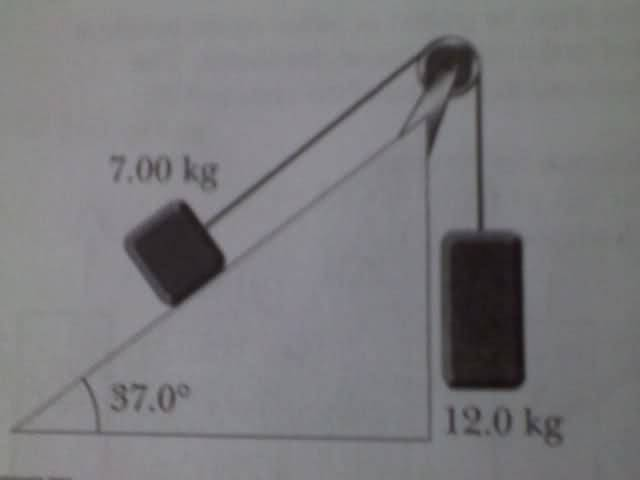# Basic Newton's Law Problem, 2 Blocks on a frictionless ramp/pulley

• ihatephysicss

## Homework Statement

Draw a free body diagram for the block on the frictionless ramp below. Break up any problem vectors into friendly vectors. Will you work in x and y components or parallel and perpendicular? Then, find the magnitude of each force you have labeled. Lastly, find the acceleration of the block down the ramp.

Repeat this problem with friction between the ramp and the block. The coefficient of friction is 0.2. What is the new acceleration of the block?Refer to this diagram ^

F=ma
F=mg

## The Attempt at a Solution

I am guessing you have to split the problem up into vectors involving triangles, and use SOHCAHTOA. (sin, cos, tan)
My answer is 0.86 m/s^2 for the acceleration, but I'm not sure this is right.

How did you get the answer? Show the FBD-s, and the calculations in detail, please.

ehild